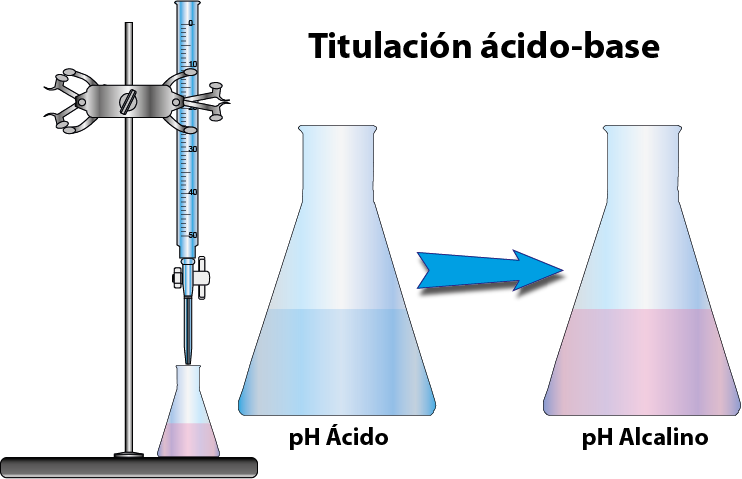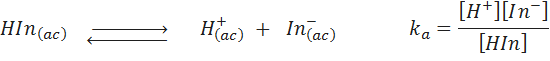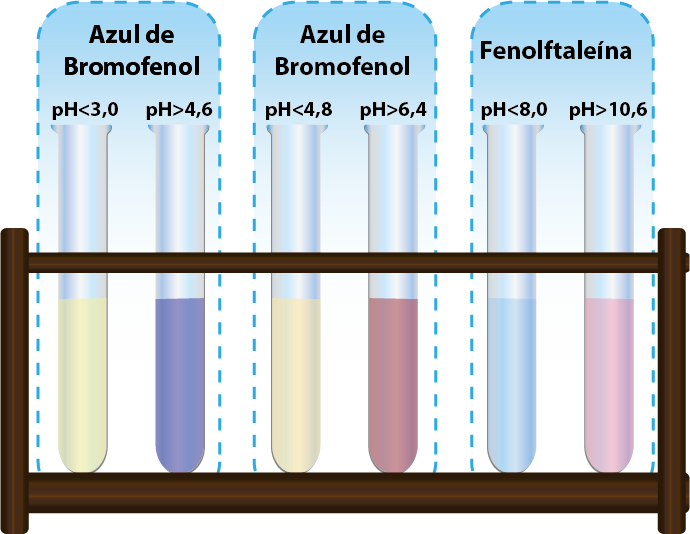HomeenWhat is an acid base indicator?

# What is an acid base indicator?

Acid-base indicators are compounds or chemicals that exhibit markedly different colors at different pH values . Although they serve to show the pH range of a solution at a glance, they are mainly used in the chemistry laboratory as a means to visualize the equivalence point during an acid-base titration or titration.

These compounds are always weak organic acids or bases that have a different color when protonated (at low pH) than when deprotonated (at high pH). They are usually monoprotic or monobasic species, which means that they are only involved in a single ionic equilibrium and exhibit a single color change.

Some typical examples of acid base indicators are phenolphthalein, methyl red and thymol blue. Homemade acid-base indicators such as red cabbage extract (red cabbage) can also be prepared. This one can be made by simply boiling a red cabbage in water for a few minutes. The resulting solution will present a series of colors at different pH ranging from red, purple, deep green to yellow.

The concept of acid-base indicator is closely linked to acid-base titrations and to two other related concepts, the equivalence point and the end point . Understanding what each one is about will allow you to better understand what acid base indicators are and what they are for.

## Acid Base Titrations

Acid-base titrations are analytical techniques that chemists use to determine the concentration of an acid or base (called an analyte) in an unknown sample . To do this, an aliquot of the sample to be analyzed is taken and a solution of a base or an acid of known concentration (called titrant ) is added drop by drop until the analyte is completely neutralized.The exact point at which the analyte is neutralized is called the equivalence point , and the volume of the titrant required to reach that point together with its concentration allow the concentration of the analyte in the sample to be determined.

The problem with titrations is that the analytical chemist carrying out the titration has no way of telling when the equivalence point has been reached. Acid-base indicators add to the analyte as they undergo a visible color change upon reaching or exceeding the equivalence point.

## The end point versus the equivalence point

As mentioned above, indicators are primarily used to display or “indicate” the equivalence point during an acid-base titration. However, depending on the acidity or basicity constant of the analyte, such an equivalence point will occur at different pH values, and this pH rarely exactly coincides with the pH at which an indicator changes color. Despite this, the color change in the indicator is the only reference that the chemist can use to know that it is time to stop the titration. For this reason, chemists refer to the point at which an indicator changes color as the “end point,” to distinguish it from the true “equivalence point” they are looking for.

The ideal acid-base indicator is one that shows a strong color change exactly at the pH of the equivalence point of the titration. In other words, it is the one with which the end point coincides exactly with the equivalence point. Unfortunately, the ideal acid-base indicator does not exist, but there are different indicators that have different pH ranges at which they turn or change color. This is usually enough to get a good approximation of the equivalence point without making a very large experimental error.

For this reason, whenever an acid-base titration is going to be carried out, one should start by choosing the indicator that changes color as close as possible to the pH of the equivalence point of the analyte in question.

## Operation of the Acid Base Indicators

Acid-base indicators are weak acids or bases that are involved in an acid-base equilibrium between their protonated acid form and their conjugate base or deprotonated form. Those that are weak acids are neutral in acidic pH and negative in alkaline pH, and the opposite happens to those that are weak bases, that is, they are neutral in alkaline pH and positive ions in acidic pH.

To understand why this happens, let’s consider the equilibrium in which an acid-base indicator is involved. The reaction can be represented as follows:Here, k a represents the acid dissociation constant of the indicator.

Based on Le Chatelier’s principles, when the concentration of H + ions is high, that is, when the pH is low, this equilibrium shifts to the left, that is, towards the protonated form HIn. In this case, we see the color of HIn because it is the species that is present in the highest proportion. When the opposite occurs (at low H + concentration ), the equilibrium shifts towards the products. In this case, the most abundant species whose color we see with the naked eye is now In – .

Normally, we are able to distinguish one color or the other when the concentration of one is more than 10 times greater than that of the other.

## Examples of acid base indicators

The following table shows some examples of acid-base indicators commonly used in the chemistry laboratory, ordered according to the pH range in which their color change occurs.

Indicator Color in acid medium Color in alkaline medium End Point pH Range thymol blue Red Yellow 1.2 – 2.8 bromophenol blue Yellow bluish purple 3.0 – 4.6 Methyl orange Orange Yellow 3.1 – 4.4 Methyl red Red Yellow 4.2 – 6.3 chlorophenol blue Yellow Red 4.8 – 6.4 bromothymol blue Yellow Blue 6.0 – 7.6 cresol red Yellow Red 7.2 – 8.8 phenolphthalein Colorless Pinkish 8.3 – 10.0These pH ranges can be determined using the acidity constant of the indicator and calculating the pH at which [HIn]/[In – ]≥10 (when there are ten times more HIn than In – ) and when [HIn]/ [In – ]≤0.1 (when there are ten times more In – than HIn).

## How do you select the right acid base indicator?

In general, if you plan to titrate to a strong acid or strong base, almost any indicator can be used, since in such titrations the pH changes dramatically from very acid to very basic or from very basic to very acid just before and after the titration. equivalence point, which occurs exactly at pH = 7.

On the other hand, if you are titrating a weak acid or base, you should start by looking at its acidity constant, or, more precisely, the pKa of the analyte (pKa is the negative of the logarithm of the equilibrium constant). . This value represents the pH of the solution halfway to the equivalence point, and provides a clue as to the pH at which the equivalence point will be reached. Since the sample has an unknown concentration (since the titration is performed just to find its concentration), we can’t exactly calculate the pH of the equivalence point, but the pKa gives us a general idea of ​​where it is on the pH scale. pH.

Thus, the appropriate indicator will be the one whose color change pH range is as centered as possible on said pKa.

### Example of selection of an acid base indicator

• When titrating strong acids or bases whose equivalence point occurs at pH=7, phenolphthalein is almost always used, even though it changes color between 8.3 and 10.0. One could also select bromothymol blue or cresol red, whose turns are much closer to pH=7, but in general it is not necessary.
• If you want to titrate a weak acid with a pKa of 3.9 by adding NaOH (which is a strong base) then you could select methyl orange, since it changes color between pH=3.1 and pH=4.4, or methyl red, which changes to a slightly more alkaline pH. Remember that at pH = 3.9 (that is, when pH=pKa), the equivalence point has not yet been reached, so you have to keep adding more NaOH, so the equivalence point will be at a higher pH. than 3.9.